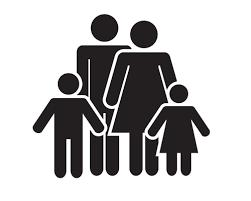# Father 4510

The father is 48 years old, and the son is 21. How many years ago was the father ten times older than the son?

x =  18

### Step-by-step explanation:

48-x = 10(21-x)

48-x = 10·(21-x)

9x = 162

x = 162/9 = 18

x = 18

Our simple equation calculator calculates it.Did you find an error or inaccuracy? Feel free to write us. Thank you!

Tips for related online calculators
Do you have a linear equation or system of equations and looking for its solution? Or do you have a quadratic equation?
Do you want to convert time units like minutes to seconds?